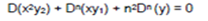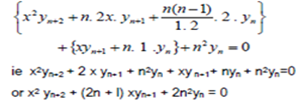Problems on Leibnitz Theorem-2: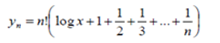10. If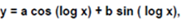show that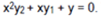Then apply Lebnitz theorem to differentiate or,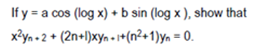Solutions: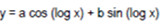Differentiate w.r.t x we get;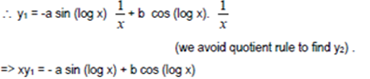Differentiate again w.r.t. x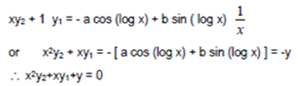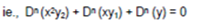Apply Lebnitz we have;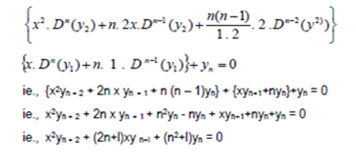11. If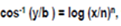Then show that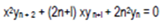Solutions: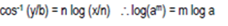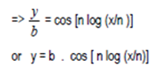Differentiate it to n times we get;